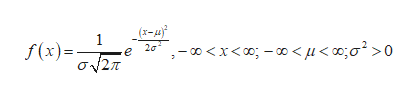In its Fuel Economy Guide for 2016 model vehicles, the Environmental Protection Agency provides data on 1170 vehicles. There are a number of high outliers, mainly hybrid gas‑electric vehicles. If we ignore the vehicles identified as outliers, however, the combined city and highway gas mileage of the other 1146 vehicles is approximately Normal with mean 23.0 miles per gallon (mpg) and standard deviation 4.9 mpg.The quartiles of any distribution are the values with cumulative proportions 0.25 and 0.75. They span the middle half of the distribution.What is the first quartile of the distribution of gas mileage?  give your answer rounded to two decimal places. What is the third quartile of the distribution of gas mileage?give your answer rounded to two decimal places.

Question

In its Fuel Economy Guide for 2016 model vehicles, the Environmental Protection Agency provides data on 1170 vehicles. There are a number of high outliers, mainly hybrid gas‑electric vehicles. If we ignore the vehicles identified as outliers, however, the combined city and highway gas mileage of the other 1146 vehicles is approximately Normal with mean 23.0 miles per gallon (mpg) and standard deviation 4.9 mpg.

The quartiles of any distribution are the values with cumulative proportions 0.25 and 0.75. They span the middle half of the distribution.

What is the first quartile of the distribution of gas mileage?  give your answer rounded to two decimal places.

What is the third quartile of the distribution of gas mileage?give your answer rounded to two decimal places.

Step 1

The Normal distribution:

A continuous random variable X is said to follow normal distribution with mean µ and standard deviation σ if the probability density function of X is,help_outlineImage Transcriptionclose(x- 2a 00x 1 f(x)=- 12 o < pu < 0o>0 fullscreen
Step 2

Quartiles:

Three Quartiles,

• The first quartile separates the lowest 25% of the observations from the higher 75% of the observations. The first quartile is denoted by Q1.
• The second quartile separates the lower 50% of the observations from the higher 50% of the observations. The second quartile is denoted by Q2.The second quartile is same as median.
• The third quartile separates the lowest 75% of the observations from the higher 25% of the observations. The third quartile is denoted by Q3.

Step 3

Computing the first quartile of the distribution of gas mileage:

The first quartile is the x-value s...

Want to see the full answer?

See Solution

Want to see this answer and more?

Our solutions are written by experts, many with advanced degrees, and available 24/7

See Solution
Tagged in

Statistics